http://people.csail.mit.edu/jaffer/CNS/PSFCDR

# The Performance of Space-Filling Curves for Dimension Reduction

## Motivation

Color Space Dimension Reduction explores methods of dimension reduction, focusing on space-filling curves. For the purpose of presenting colors for selection, the space-filling curve attributed to Hilbert seems to perform better than the curve due to Peano. Can that difference be quantified?

The goal of dimension reduction is to find a mapping from a higher-dimensional space to a lower-dimensional space where points which are close in the higher-dimensional space map to points which are close in the other space.

## Apparatus

• 2009-06-10 Development version of SCM 5e5
• 2009-06-10 Development version of SLIB 3b1
• Scheme program closeness.scm
• the "convert" program, part of ImageMagick-6.3.2

## Procedure

• `scm -l closeness.scm`
• Graphs are written in the working directory:
"Z-curve-r.png"
Graph of Euclidean-distance / scalar-distance versus the scalar-distance between two points for the Z-curve in 2, 3, 4, and 6 dimensions.
"Z-curve-l.png"
Graph of the logarithm of Euclidean-distance versus the logarithm of the scalar-distance between two points for the Z-curve in 2, 3, 4, and 6 dimensions.
"Peano-r.png"
Graph of Euclidean-distance / scalar-distance versus the scalar-distance between two points for the Peano space-filling curve in 2, 3, 4, and 6 dimensions.
"Peano-l.png"
Graph of the logarithm of Euclidean-distance versus the logarithm of the scalar-distance between two points for the Peano space-filling curve in 2, 3, 4, and 6 dimensions.
"Hilbert-r.png"
Graph of Euclidean-distance / scalar-distance versus the scalar-distance between two points for the Hilbert space-filling curve in 2, 3, 4, and 6 dimensions.
"Hilbert-l.png"
Graph of the logarithm of Euclidean-distance versus the logarithm of the scalar-distance between two points for the Hilbert space-filling curve in 2, 3, 4, and 6 dimensions.

## Method

• The closeness.scm program fills an array with the multidimensional coordinates corresponding to 4096 (or 729 for Peano curves) successive integers.
• For a given dimension it then computes, for each scalar-distance from 1 to 15, the average ratio of Euclidean-distance to the scalar-distance for all pairs of points having that scalar separation.
• The resulting graphs are written to graphic files:
• "Z-curve-r.png"
• "Peano-r.png"
• "Hilbert-r.png"
• For a given dimension it then computes, for each scalar-distance from 1 to 100, the logarithm of the average Euclidean-distance for all pairs of points having that scalar separation.
• The resulting graphs are written to graphic files:
• "Z-curve-l.png"
• "Peano-l.png"
• "Hilbert-l.png"

## Measurements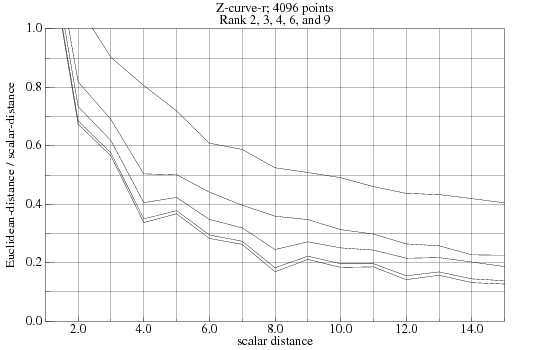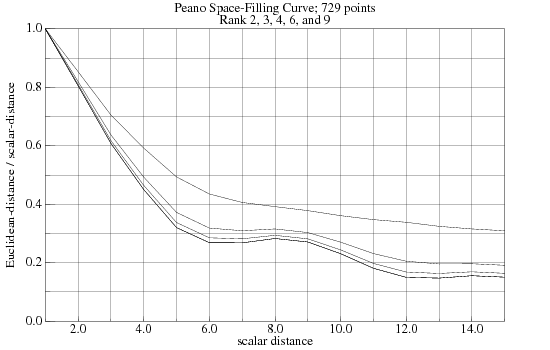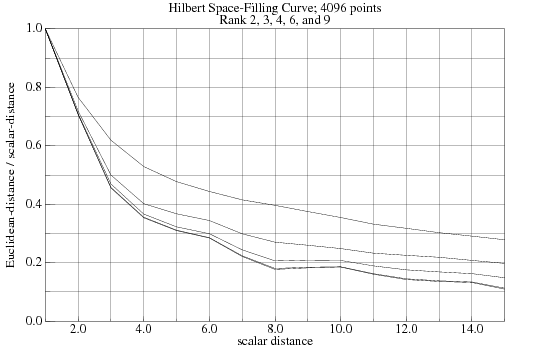## AnalysisThe Z-curve has the widest spread and is particularly poor in 2-dimensions (the top plot). Worse still is that most unit length scalar edges map to coordinates which are more than one unit separated. Thus the average displacement for unit edges averages above 1.6 for 2-dimensions and above 1.35 for the other ranks.

The ratios for all three functions quickly approach their low, asymptotic values as the rank is increased. For the Peano and Hilbert curves the traces for ranks 6 and 9 are coincident.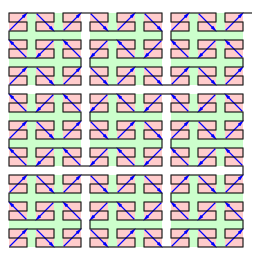The space-filling curve attributed to Peano maps scalar unit lengths to unit Euclidean lengths, giving optimal behavior for the shortest length. In fact all horizontal segments are exactly unit length. The vertical segments run 2 and 5 units; raising the averages in that range.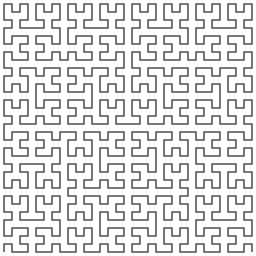The space-filling curve attributed to Hilbert edges out the Peano curve to have the best performance of those measured. Color-Space Dimension Reduction also finds the Hilbert curve (slightly) better than Peano's. And [Bongki 2001] rates it best as well.

## Logarithmic Measurements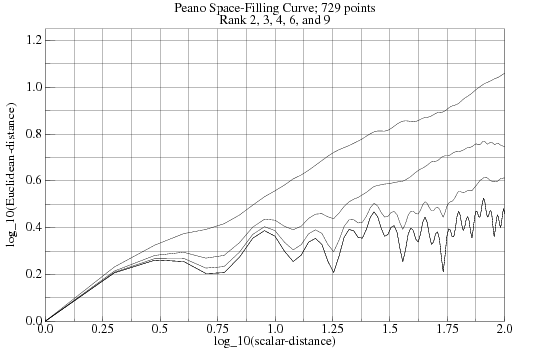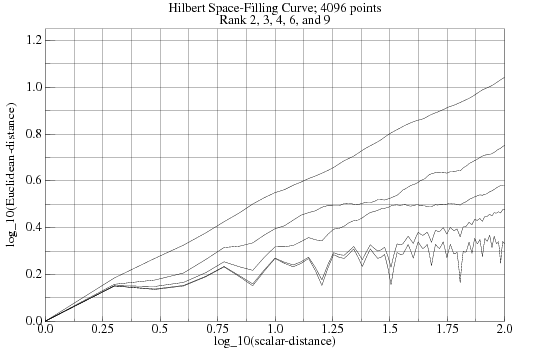Plotting the (logarithm of) average Euclidean-distance versus the (logarithm of) scalar-distance out to longer distances seems to indicate that the roughness of the high rank curves does not become smooth asymptotically.